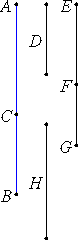# Proposition 51

To find the fourth binomial straight line.

Set out two numbers AC and CB such that AB has neither to BC nor to AC the ratio which a square number has to a square number.

Set out a rational straight line D, and let EF be commensurable in length with D. Then EF is also rational.Let it be contrived that the number BA is to AC as the square on EF is to the square on FG. Then the square on EF is commensurable with the square on FG. Therefore FG is also rational.

X.9

Now, since BA does not have to AC the ratio which a square number has to a square number, neither does the square on EF have to the square on FG the ratio which a square number has to a square number, therefore EF is incommensurable in length with FG.

Therefore EF and FG are rational straight lines commensurable in square only, so that EG is binomial.

I say next that it is also a fourth binomial straight line.

Since BA is to AC as the square on EF is to the square on FG, therefore the square on EF is greater than the square on FG.

V.19,Cor.

Let then the sum of the squares on FG and H equal the square on EF. Then, in conversion, the number AB is to BC as the square on EF is to the square on H.

But AB does not have to BC the ratio which a square number has to a square number, therefore neither does the square on EF have to the square on H the ratio which a square number has to a square number.

X.9

Therefore EF is incommensurable in length with H. Therefore the square on EF is greater than the square on GF by the square on a straight line incommensurable with EF.

And EF and FG are rational straight lines commensurable in square only, and EF is commensurable in length with D. Therefore EG is a fourth binomial straight line.

Q.E.D.

## Guide

This proposition is not used in the rest of the Elements.